##### The Japanese Bonsai specialist
Direct order Contact Help / Services Newsletter# Pinus pentaphylla 'shoon's bonsai'

Pinus pentaphylla "blue lou"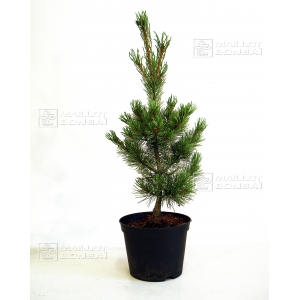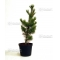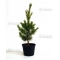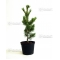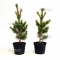ref. : 1144118,00

This item is temporarily unavailableNotify availability

###### Description

Tree produced by grafting.

Size 35/40 cm.

Sold in a 5 litres pot.

A dwarf variety, pinus pentaphylla 'shoon's bonsai' is an ideal species for creating small to mid-sized bonsai. The needles are very small, appearing green in the spring and blue all summer, resulting in the most beautiful effect. We recommend cleaning it annually, getting rid of any dead needles on the branches.

Likes sun/mid-shade and fertilising with Biogold once a month until the end of October.

#bonsai 4.6 #pentaphylla 4.1 #shoon 3.5 #pinus 3.5 #needles 2.7 #young 2.5 #trees 2.5 #small 2.5 #fertilising 2.1 #beautiful 1.9

Formule
(( ROUND((CHAR_LENGTH(b.article_nom)-CHAR_LENGTH(REPLACE(b.article_nom, 'bonsai', '')))/LENGTH('bonsai')) + ROUND((CHAR_LENGTH(b.article_description)-CHAR_LENGTH(REPLACE(b.article_description, 'bonsai', '')))/LENGTH('bonsai')) ) * 4.6) + (( ROUND((CHAR_LENGTH(b.article_nom)-CHAR_LENGTH(REPLACE(b.article_nom, 'pentaphylla', '')))/LENGTH('pentaphylla')) + ROUND((CHAR_LENGTH(b.article_description)-CHAR_LENGTH(REPLACE(b.article_description, 'pentaphylla', '')))/LENGTH('pentaphylla')) ) * 4.1) + (( ROUND((CHAR_LENGTH(b.article_nom)-CHAR_LENGTH(REPLACE(b.article_nom, 'shoon', '')))/LENGTH('shoon')) + ROUND((CHAR_LENGTH(b.article_description)-CHAR_LENGTH(REPLACE(b.article_description, 'shoon', '')))/LENGTH('shoon')) ) * 3.5) + (( ROUND((CHAR_LENGTH(b.article_nom)-CHAR_LENGTH(REPLACE(b.article_nom, 'pinus', '')))/LENGTH('pinus')) + ROUND((CHAR_LENGTH(b.article_description)-CHAR_LENGTH(REPLACE(b.article_description, 'pinus', '')))/LENGTH('pinus')) ) * 3.5) + (( ROUND((CHAR_LENGTH(b.article_nom)-CHAR_LENGTH(REPLACE(b.article_nom, 'needles', '')))/LENGTH('needles')) + ROUND((CHAR_LENGTH(b.article_description)-CHAR_LENGTH(REPLACE(b.article_description, 'needles', '')))/LENGTH('needles')) ) * 2.7) + (( ROUND((CHAR_LENGTH(b.article_nom)-CHAR_LENGTH(REPLACE(b.article_nom, 'small', '')))/LENGTH('small')) + ROUND((CHAR_LENGTH(b.article_description)-CHAR_LENGTH(REPLACE(b.article_description, 'small', '')))/LENGTH('small')) ) * 2.5) + (( ROUND((CHAR_LENGTH(b.article_nom)-CHAR_LENGTH(REPLACE(b.article_nom, 'fertilising', '')))/LENGTH('fertilising')) + ROUND((CHAR_LENGTH(b.article_description)-CHAR_LENGTH(REPLACE(b.article_description, 'fertilising', '')))/LENGTH('fertilising')) ) * 2.1) + (( ROUND((CHAR_LENGTH(b.article_nom)-CHAR_LENGTH(REPLACE(b.article_nom, 'beautiful', '')))/LENGTH('beautiful')) + ROUND((CHAR_LENGTH(b.article_description)-CHAR_LENGTH(REPLACE(b.article_description, 'beautiful', '')))/LENGTH('beautiful')) ) * 1.9) + (( ROUND((CHAR_LENGTH(b.article_nom)-CHAR_LENGTH(REPLACE(b.article_nom, 'resulting', '')))/LENGTH('resulting')) + ROUND((CHAR_LENGTH(b.article_description)-CHAR_LENGTH(REPLACE(b.article_description, 'resulting', '')))/LENGTH('resulting')) ) * 1.9) + (( ROUND((CHAR_LENGTH(b.article_nom)-CHAR_LENGTH(REPLACE(b.article_nom, 'recommend', '')))/LENGTH('recommend')) + ROUND((CHAR_LENGTH(b.article_description)-CHAR_LENGTH(REPLACE(b.article_description, 'recommend', '')))/LENGTH('recommend')) ) * 1.9)

## Secure payment## Delivery

Our logistic partners :04 74 55 23 48
Pépinière MAILLOT-BONSAÏ
Le Bois Frazy
01990 RELEVANT - FRANCE
on appointment